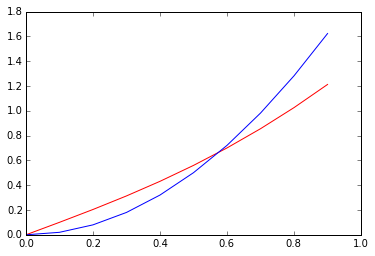# 大O记法

## 大O记法（Big-O Notation）

    x = range(1,7)

factorial = [np.math.factorial(i) for i in x]
exponential = [np.e**i for i in x]
polynomial = [i**3 for i in x]
logarithmic = [np.log(i) for i in x]

plt.plot(x,factorial,'black',\
x,exponential, 'blue',\
x,polynomial, 'green',\
x,logarithmic, 'red')from sympy.abc import x
# sympy中无限infty用oo表示
print ((sympy.E**x)/(x**3)).limit(x,sympy.oo)
# result is: oo
print (sympy.ln(x)/x**3).limit(x,sympy.oo)
# result is 0


    xvals = np.linspace(0,100,1000)
f = x*sympy.sqrt(1+x**2)
g = 2*x**2
y1 = [f.evalf(subs={x:xval}) for xval in xvals]
y2 = [g.evalf(subs={x:xval}) for xval in xvals]
plt.plot(xvals[:100],y1[:100],'r',xvals[:100],y2[:100],'b')
plt.plot(xvals,y1,'r',xvals,y2,'b')Sympy可以帮助我们分析一个函数的阶：

    print sympy.O(f, (x, sympy.oo))
# result is : O(x**2, (x, oo))


    print sympy.order(f, (x, 0))
# result is : O(x)


## 误差分析（Error Analysis）

    exp = e**x
sum15 = exp.series(x,0,15).removeO()


    print exp.series(x, 0, 3)
# result is : 1 + 1.0*x + 0.5*x**2 + O(x**3)


{% math %}cos(x^2)\sqrt{(x)}=(1-\frac{1}{2}x^4+O(x^6))x^{\frac{1}{2}}{% endmath %}
{% math %}\qquad = x^{\frac{1}{2}}- \frac{1}{2}x^{\frac{9}{2}} + O(x^{\frac{13}{2}}){% endmath %}

    print (sympy.cos(x**2)*sympy.sqrt(x)).series(x,0,5)
# result is : sqrt(x) - x**(9/2)/2 + O(x**5)